## Topic 6.3: Transformations to Linear Regression (Engineering Application)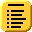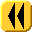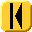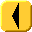Introduction Notes Theory HOWTO Examples Engineering Error Questions Matlab Maple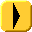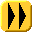The response of any linear system may be shown to have exponential responses when a system has zero input. For example, the charge on a capacitor decreases exponentially when placed in a loop together with a resistor.

It is possible to estimate the rate at which a capacitor is discharging by sampling the voltage across the capacitor periodically, and since it is known that the capacitor discharges exponentially, it would make more sense to try to fit the data points to an exponential function than it would be to try to use polynomial interpolation.

# Power Transformation

Asymptotic run times may be determined with power transformations: data which is believed to run in Θ(nk) can be tested by finding the best-fitting line which passes through the points (ln(x1), ln(y1)), ..., (ln(xn), ln(yn)) and the slope of that line will approximate k.

This works whether n → ∞ (for example, an algorithm designed to work with a problem of size n) or n → 0 (for example, a numerical methods algorithm). We will use the power transformation to show that future methods converge as claimed.